React Trash Collector State Machine

My brother gave me a problem to solve, he said that in Sean Carrol book Something Deeply Hidden he talks about a problem where a robot has to collect trashes in a 2D grid.

However the Robot can only see the top, down, left and right grid around it, moreover he has a short memory, he forgets about the past state (stateless) every time he perform a action, that could be, move left, right, up, down or get the trash.

The question is, what is the best set of action mapped to state that would get the minimum amount of moves and collect all trashes in a random grid size on average?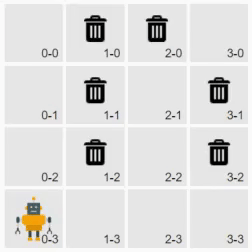This problem can be define in a state machine model where the robot can be in 162 different states.

const SURROUND_GRID_POSITION = ['up, 'down', 'left', 'right', 'current'].length
const GRID = ['empty', 'wall', 'trash'].length
SURROUND_GRID_POSITION ** GRID === 162

However 25 are not possibles since is a 2D grid and some configuration, as been surround by wall are not possible.

State Machine Diagram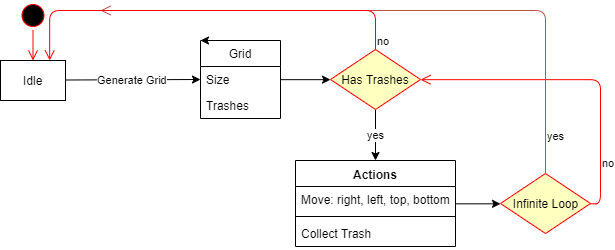An nothing better than React from dealing with states.

For generating a new 2D grid with size and trash chance per grid and return a tuple [grid: Grid, robotPos: number[]], and grid been a nested array with [pos: number[], hasTrash: boolean, hasRobot: boolean], so we can map inside React.

export function generateGridArray(size, chanceOfTrash) {
let robotPos;
const genRobotPos = () => {
const posX = Math.floor(Math.random() * size);
const posY = Math.floor(Math.random() * size);
robotPos = [posX, posY];
//compare pos
return (x, y) => (posX === x && posY === y ? true : false);
};
const checkPos = genRobotPos();
// generate 2D grid
const grid = [];
for (let x = 0; x < size; x++) {
const boxes = [];
for (let y = 0; y < size; y++) {
const pos = [x, y];
const hasTrash = Math.random() * 100 < chanceOfTrash ? true : false;
const hasRobot = checkPos(x, y);
const oldPos= checkPos(x,y)
boxs.push([pos, hasTrash, hasRobot]);
}
grid.push(boxes);
}
return [grid, robotPos];
}

In order for a O(1) lookup we should have a unique value for the 137 possible states and create a Object representing the state with the equivalent action:

export const stateValues = {
left: { trash: 2, empty: 3, wall: 5 },
right: { trash: 7, empty: 11, wall: 13 },
up: { trash: 17, empty: 19, wall: 23 },
down: { trash: 29, empty: 31, wall: 37 },
current: { trash: 41, empty: 43 }
};

function getGridArrayStateSum(gridArray) {
//return a unique gridArray value that represent the state grid
let stateSum = 0;
let robotPos = []
let boxNum = 1;
for (let i = 0; i < gridArray.length; i++) {
for (let j = 0; j < gridArray[i].length; j++) {
let box = gridArray[i][j];
if (box){ // has trash
stateSum += boxNum * (gridArray.length ** 2)
}
if (box){ // has robot
robotPos = boxNum
}

boxNum++;
}
}
return [stateSum, robotPos]
}

Another helper function is to check if the game is over or if the Robot got into a infinite loop, by saving the passed states.

function gameOver(gridArray) {
return !gridArray.some(arr => arr.some(e => e === true));
}

export function infinitLoop() {
let passedState = {};

return function(gridArray) {
// robotBoxnum is the position of the robot in the grid starting at 1
let [currentStateSum, robotBoxNum] = getGridArrayStateSum(gridArray)

let trashStates = passedState[robotBoxNum]

if (!trashStates){ // if no passed state in robot location
passedState[robotBoxNum] = [currentStateSum] // add new location with trash sum
}else{ // if robot been in passed location
if (trashStates.includes(currentStateSum)){ // check if trash sum already in the location
return true  // robot been save position with same trash configuration

}else {
passedState[robotBoxNum].push(currentStateSum)
}
}
return false
};
}

Also the default game logic will move the Robot randomly to a grid if has a trash on it, get the trash if its in current position of the Robot or move to a random grid, behaving as so: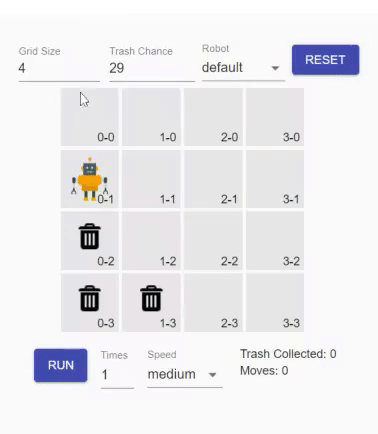Intuitively I supposed that the best behavior to collect all trashes in a random Grid with the minimum amount of moves, on average, would be the default game logic, and I need tools in order to test this hypothesis. For doing that I need to map my custom action, for each state, and score the efficiency of each state. Doing in a reducer fashion way, so I can have access in all components later:

const {state, dispatch} = useContext(Pcontext);

function mapStateAction (newAction){
let newPayloadState = {[stateSum]: newAction }
}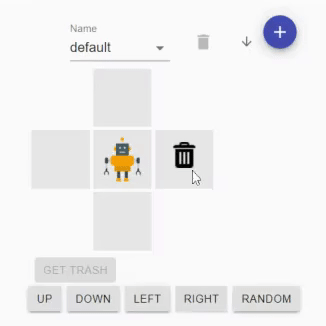But I need also a way to generate new Robots, test it and quantify its result, and for that a simple table that can compute the average for each game would be enough.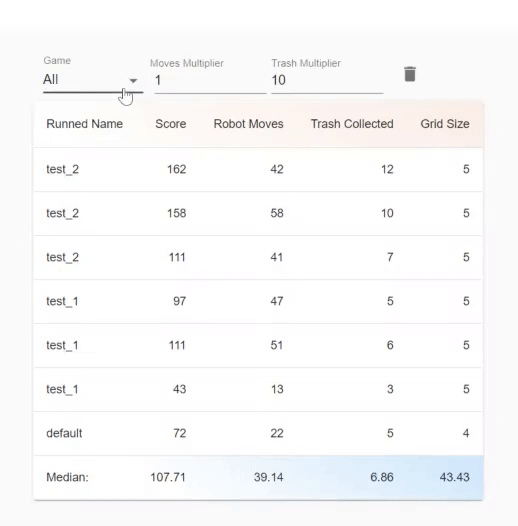However there's too many different states, for a 5x5 grid size there's is 3400 different possible Robots. I need a way to generate random Robots and select the best ones.

And for that I need many states:

const [sampleSize, setSampleSize] = useState(10)
const [selectionPercetage, setSelectionPercetage] = useState(30)
const [iteration, setIteration] = useState(30)
const [robots, setRobots] = useState(null)
const [movesMultiplier, setMovesMultiplier] = useState(1)
const [trashCollectedMultiplier, setTrashCollectedMultiplier] = useState(10)
const [displayGrid, setDisplayGrid] = useState(false)
const [running, setRunning] = useState(false)
const [gridSize, setGridSize] = useState(5)
const [trashChange, setTrashChange] = useState(20)
const [gridArray, setGridArray] = useState(null)

And now, I need to generate an array of random robots that only do possible action in the Grid, run them in on the grid and save the total of moves, size of grid, and total trashes collected and compute a score.

Since JavaScript Objects are assign by reference, and I need a new Grid Object for each Robot iteration the only way I found to make React update the Grid was by transforming in a string and parse back to object, probably not the best way ...

JSON.parse(JSON.stringify(gridArray))

I have all my tools in order to test many iteration and select the one which has the higher score by the top selection percentage * sample size of each sample, and add to the new iteration, N iteration time.

let selectedRobots = []
robots.sort((a, b) => b.score - a.score)
for (let robot of robots) {
if (selectedRobots.length < selectionNumber){
selectedRobots.push(robot)
}
}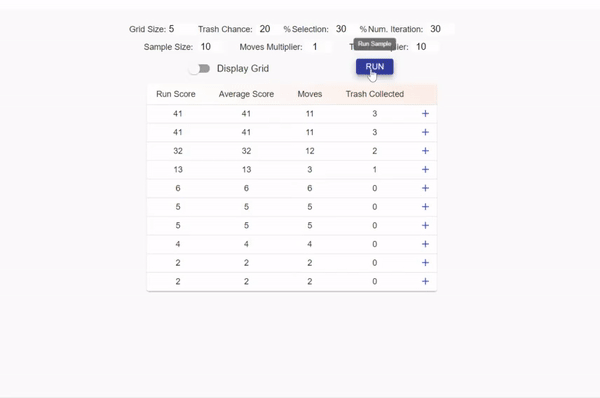After trying some huge iteration (10^5), that took some time ... I think I found my answer for the question, however I wont give any spoiler, be welcome to try for yourself at:

trashcollectorrobot.netlify.com